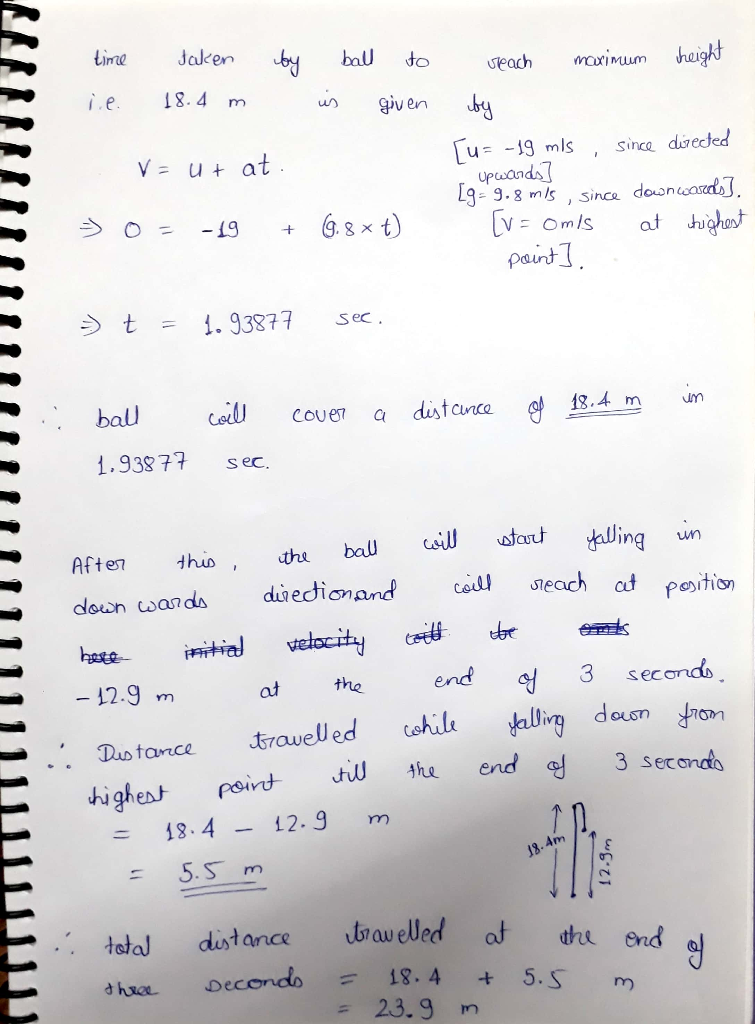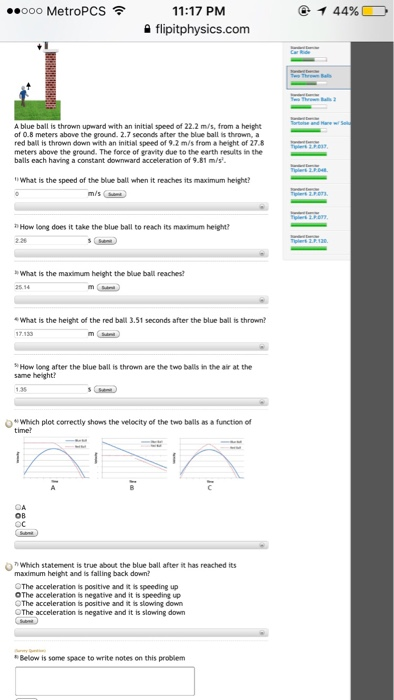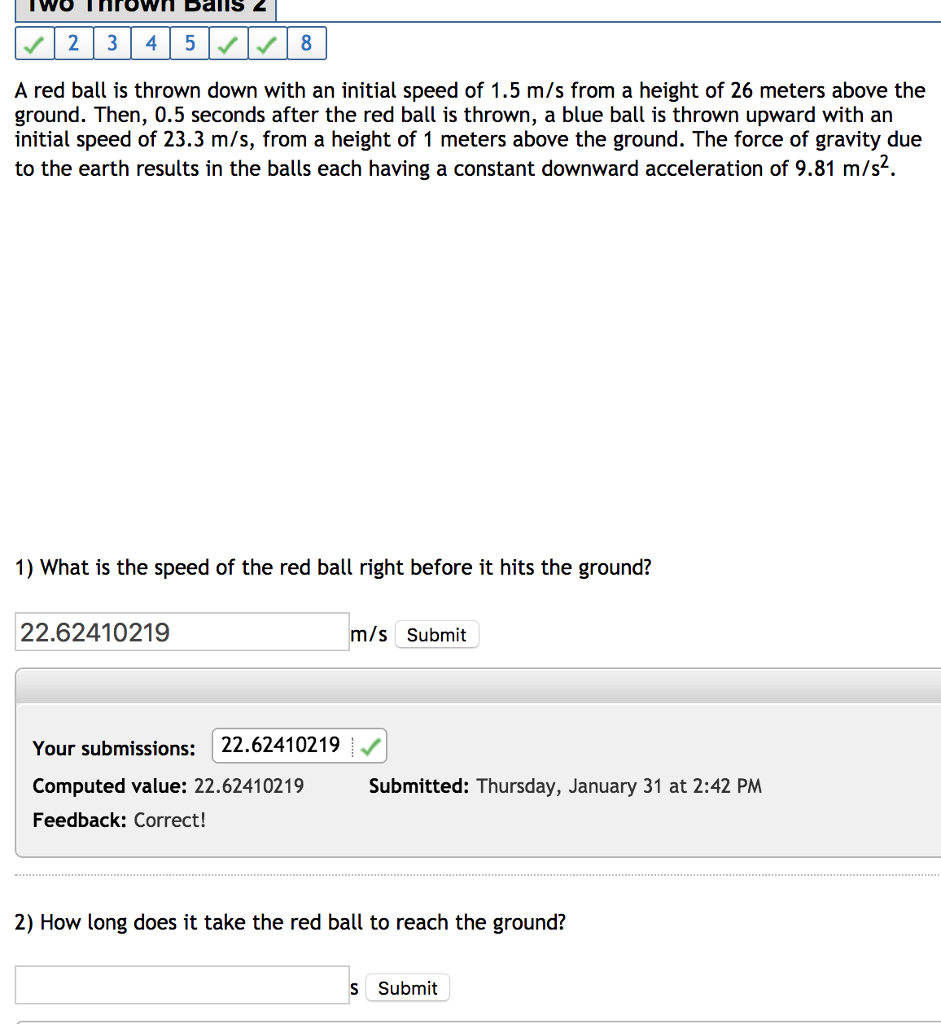# A ball is thrown upwards with a speed of 19 m/s. it reaches a hight of...

A ball is thrown upwards with a speed of 19 m/s. it reaches a hight of 18.4 m above the point of release before it falls back down. Specify down as the positive direction and the initial position as the origin. If the balls position after three seconds is -12.9 meters, what is the distance traveled by the rock? The acceleration due to gravity is 9.8 m/s^2 down. The answer is 23.9 meters, how would i solve this?#### Earn Coin

Coins can be redeemed for fabulous gifts.

Similar Homework Help Questions
• ### A ball is thrown upwards with a speed of 19 m/s. it reaches a hight of 18.4 m above the point of release before it falls...

A ball is thrown upwards with a speed of 19 m/s. it reaches a hight of 18.4 m above the point of release before it falls back down. Specify down as the positive direction and the initial position as the origin. If the balls position after three seconds is -12.9 meters, what is the distance traveled by the rock? The acceleration due to gravity is 9.8 m/s^2 down. The answer is 23.9 meters, how would i solve this?

• ### A blue ball is thrown upward with an initial speed of 23.9 m/s, from a height...

A blue ball is thrown upward with an initial speed of 23.9 m/s, from a height of 0.5 meters above the ground. 2.9 seconds after the blue ball is thrown, a red ball is thrown down with an initial speed of 7.5 m/s from a height of 31.5 meters above the ground. The force of gravity due to the earth results in the balls each having a constant downward acceleration of 9.81 m/s^2. How long after the blue ball is...

• ### Two throw A blue ball is thrown upward with an initial speed of 22.2 m/s, from...Two throw A blue ball is thrown upward with an initial speed of 22.2 m/s, from a height of 0.8 meters above the ground. 2.7 seconds after the blue ball is thrown, a red ball is thrown down with an initial speed of 9.2 m/s from a height of 27.8 meters above the ground. The force of grandly due to the earth results in the balls each having a constant downward acceleration of 9.81 m/s^2. What is the speed of...

• ### A blue ball is thrown upward with an initial speed of 19.8 m/s, from a height of 0.5 meters above the ground

A blue ball is thrown upward with an initial speed of 19.8 m/s, from a height of 0.5 meters above the ground. 2.4 seconds after the blue ball is thrown, a red ball is thrown down with an initial speed of 7.9 m/s from a height of 22.5 meters above the ground. The force of gravity due to the earth results in the balls each having a constant downward acceleration of 9.81 m/s2. What is the maximum height the blue...

• ### A red ball is thrown down with an initial speed of 1.2 m/s from a height...

A red ball is thrown down with an initial speed of 1.2 m/s from a height of 25 meters above the ground. Then, 0.6 seconds after the red ball is thrown, a blue ball is thrown upward with an initial speed of 23.8 m/s, from a height of 0.8 meters above the ground. The force of gravity due to the earth results in the balls each having a constant downward acceleration of 9.81 m/s^2. 1)What is the speed of the...

• ### A red ball is thrown down with an initial speed of 1.2 m/s from a height...

A red ball is thrown down with an initial speed of 1.2 m/s from a height of 26 meters above the ground. Then, 0.4 seconds after the red ball is thrown, a blue ball is thrown upward with an initial speed of 23.8 m/s, from a height of 1 meters above the ground. The force of gravity due to the earth results in the balls each having a constant downward acceleration of 9.81 m/s2. 1) What is the speed of...

• ### A blue ball is thrown upward with an initial speed of 24.1 m/s, from a height...

A blue ball is thrown upward with an initial speed of 24.1 m/s, from a height of 0.8 meters above the ground. 2.9 seconds after the blue ball is thrown, a red ball is thrown down with an initial speed of 10.4 m/s from a height of 31.4 meters above the ground. The force of gravity due to the earth results in the balls each having a constant downward acceleration of 9.81 m/s2. What is the height of the red...

• ### A blue ball is thrown upward with an initial speed of 20 m/s, from a height...

A blue ball is thrown upward with an initial speed of 20 m/s, from a height of 0.8 meters above the ground. 2.4 seconds after the blue ball is thrown, a red ball is thrown down with an initial speed of 5.3 m/s from a height of 22.4 meters above the ground. The force of gravity due to the earth results in the balls each having a constant downward acceleration of 9.81 m/s2. 1) How long after the blue ball...

• ### A ball is thrown upwards with a speed 11 m/s at an angle of 52o above...

A ball is thrown upwards with a speed 11 m/s at an angle of 52o above horizontal. How much time before it reaches the top of its flight? Answer in seconds. Neglect air friction and use g = 9.8 m/s2 if needed. Pay attention to the way the angle is defined.

• ### 2345 V8 A red ball is thrown down with an initial speed of 1.5 m/s from...2345 V8 A red ball is thrown down with an initial speed of 1.5 m/s from a height of 26 meters above the ground. Then, 0.5 seconds after the red ball is thrown, a blue ball is thrown upward with an initial speed of 23.3 m/s, from a height of 1 meters above the ground. The force of gravity due to the earth results in the balls each having a constant downward acceleration of 9.81 m/s2 1) What is the...